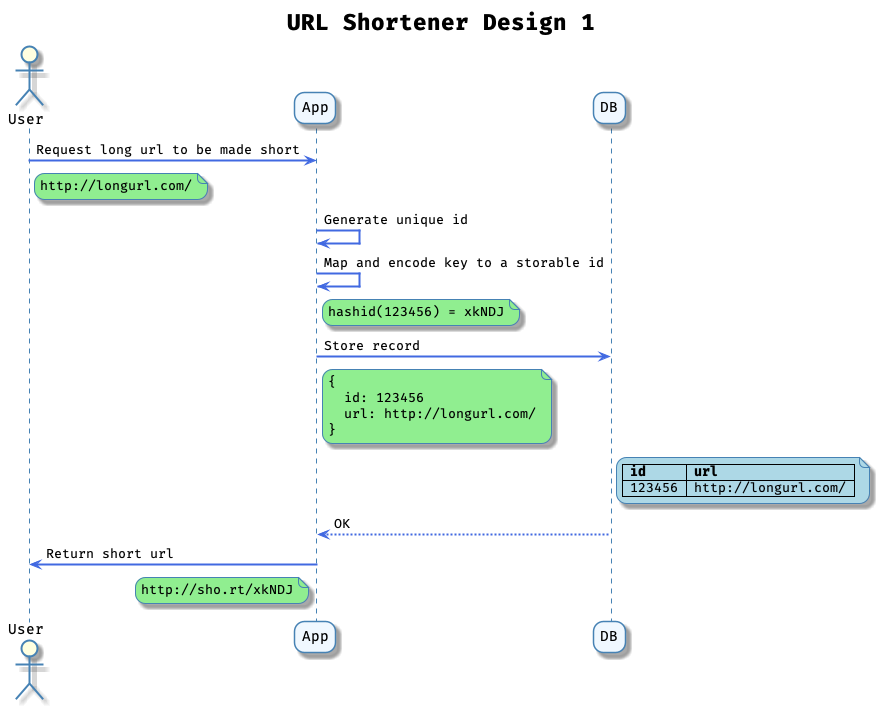# Design 1: Shared Nothing

## Analysis

Let’s start with the shared-nothing id generation (generate id in client at the App layer) since it would appear to be the most scalable.## Application design limits

We would need to generate a unique key for each request for a long url to be made short. The easiest way of doing so would be to generate an md5/sha/uuid of the long url, then we would map and encode the key and store it.

An md5/sha/uuid is $32\text{ hexchar} * 4\text{ bits/hexchar} = 128\text{ bits}$

For the best experience, we would like to have just the characters [a-z0-9] which would give us base36 encoding. If we use [a-zA-Z0-9], that would be base62 encoding, however we will be using base64 to do our estimation as $64 = 2^6$

We need to figure out the total length of the short url based on a 128 bit id.

• base2 representation, the total length would be 128. We can imagine this as a set of 128 elements each having a value of 0 or 1.
• base16 representation, a hex value is 4 bits. Each character represents $2^4 = 16$ values. We group the 128 elements into groups of 4. This would be 32 groupings, or in mathematical terms: $2^{128} = 2^{4^{32}}$. 32 hex characters is the standard representation of a uuid/md5/sha.
• base64 representation, $2^{128} = 2^{6^x}$. Taking $log_2$ of each side $(x = \dfrac{128}{6} ~= 21)$. A base64 representation would mean a 128 bit id will be represented by 21 base64 characters.

We need to make sure we store $10^{13}$ urls in the wost case of 100x traffic.

Let’s calculate the maximum address space available to us for 10 elements:

• base32: $2^{5^{10}} = 2^{10^5} = 10^{3^5} = 10^{15}$
• base64: $2^{6^{10}} = 2^{10^6} = 10^{3^6} = 10^{18}$
• $10^{13}$ urls in base32 -> $10^{13} = 2^{5^x}$. Then taking $log_2$ again, $13 * log_2(10) = 13 * 3.5 = 5 * x$ and $x \approx 9$
• $10^{13}$ urls in base64 -> $10^{13} = 2^{6^x}$. Then taking $log_2$ again, $13 * log_2(10) = 13 * 3.5 = 6 * x$ and $x \approx 8$

Given that we need about 8 characters of [a-zA-Z0-9] and 9 characters of [a-z0-9], using base32 probably provides a better user experience.

Since we calculated we only need 44 bits earlier, we can use a 64 bit key. There are other hashing functions such as murmur or fnv which are fast and support lower bit-lengths.

Let’s look at the other designs!

Previous